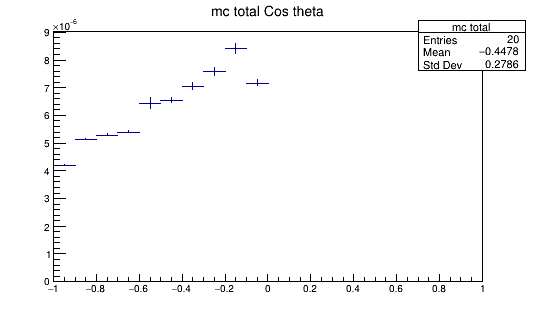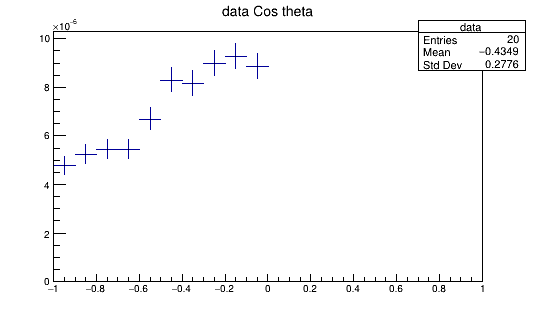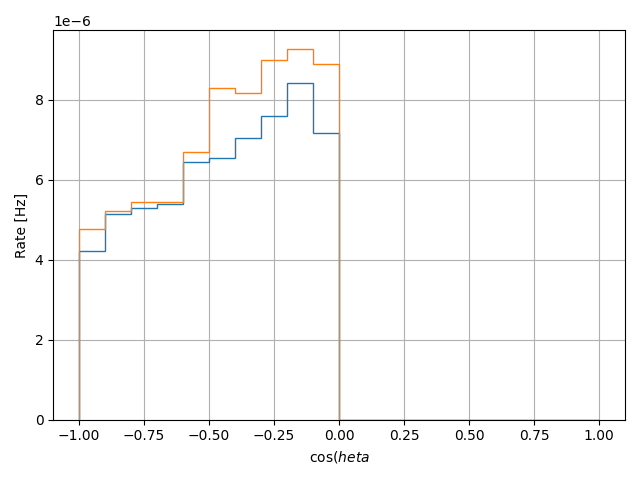# Plotting two TH1D together

Hi there!

I have a root file (“data.root”) with two TH1D files on it:

“ICRC_V2_hists/Cos theta/data” and "“ICRC_V2_hists/Cos theta/MC”I have made this script to plot the two histograms together:

import ROOT
import numpy as np
import uproot
import matplotlib.pyplot as plt

f = uproot.open(“data.root”)

fig, ax = plt.subplots()

h_data = f[“ICRC_V2_hists/Cos theta/data”].to_numpy()
h_MC = f[“ICRC_V2_hists/Cos theta/MC”].to_numpy()

hists = [h_MC, h_data]

for h in hists :

** ax.hist(h[:-1],**
** bins = h,**
** weights = h,**
** histtype = ‘step’,**
** zorder=10)**

ax.set_xlabel(‘cos(\theta’)
ax.set_ylabel(‘Rate [Hz]’)
ax.grid(zorder=0)

plt.tight_layout()
plt.show()

But the result is something like:What I want to do is to give this step format for “MC” but to keep the original dataformat for “data” (I meen, not a bar hist but points with errorbars, as seen above).

How can I do it?

h1->Draw();